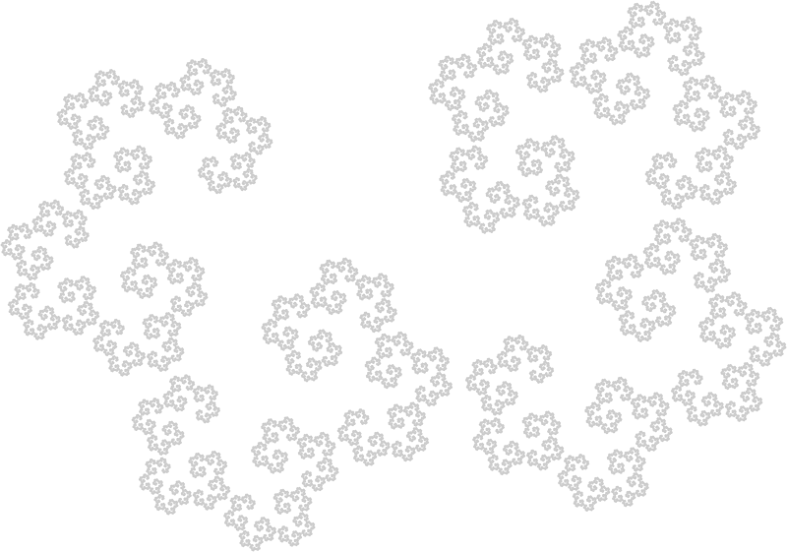## Pages

### IFS Fractals Maximize Artistic Complexity

The most fundamental aspect of creating a visual artwork, a copy of either an existing or imagined object, is that of estimating the relative proportion and angle of some part to another, whether working based on a model or from imagination. These two aspects characterize the creation of forms in the piece, as well as their overall composition. Specifically, during the creative process - as reasoned an iterative one in time - the artist uses reference points to lay out the composition, of the whole and the parts. In fact the process may be said to be fractalline, in that the artist transitions between working out global and local details, often in progressively smaller regions. Meanwhile the proportion and angular relation of reference points serves the interpolation of curves to create forms / contours. From there, the rest of the task is shading or coloring. (Though the above steps often occur in a parallel and random manner, as discussed in earlier essays.)

Therefore the artistic process hinges on the two aspects of estimating relative proportion and angle, and the following philosophical question arises: what type of object poses the greatest complexity with respect to the described creative process? We reason that it is an IFS fractal, through modelling the creative process as the "copying" of existing or imagined visual objects.A two-map IFS fractal.

Clearly an object which has infinitesimally fine features (such as a fractal), beats any other object in complexity which has none. The mathematical compactness of the object is necessary for the convergence of the iterative copying process from global to local features / regions. Partitioning these features into discrete levels from larger to smaller, in a way that the features at each level contain the next, the iterative copying occurring level-by-level. The relation of a higher level iterate to its subiterates may be assumed to be a similarity transform, possibly involving sheer. The level structure tree can be assumed to be n-ary on average and the relations to be a set of similarity IFS not involving sheer, without relevant reduction of creative complexity to be manifested via the copying process.

Therefore we have reduced the class of highest complexity to that of similarity IFS fractals with n maps. Iterating to infinity, the attractor of such an IFS - the n-map IFS fractal - is thus an object of highest possible creative complexity.

In practice, by the equation H(F)=F (where F is the IFS fractal, and H is the Hutchinson operator induced by the IFS) the task of creating / copying F via a global to local iterative process, involves copying the convex hull C (or its vertices) and making n copies Tk(C), k=1,...,n (by each IFS map Tk). Then we execute the Hutchinson H on these copies, and so on, iterating to a "high enough" level, approaching infinity. Each level being the aforementioned next level of features / regions / convex hull iterates. The challenge of complexity being the proper execution of the Hutchinson by hand drawing - proportionality and angular errors apt to accumulate, signifying the complexity.

The above argument further illustrates how a visual artwork can be characterized as the attractor of the creative process (ignoring shading / coloring), as reasoned earlier.